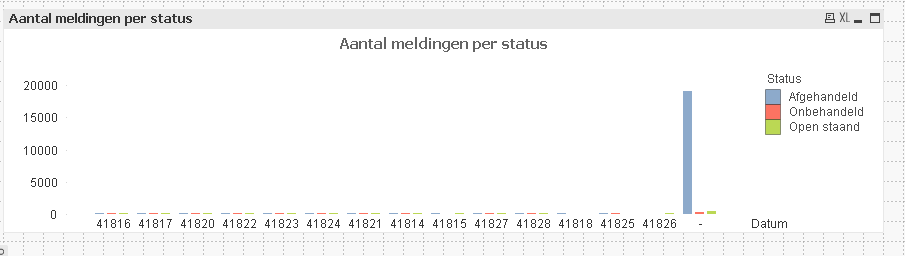# QlikView App Dev

Discussion Board for collaboration related to QlikView App Development.

Announcements
Skip the ticket, Chat with Qlik Support instead for instant assistance.
cancel
Showing results for
Did you mean:Not applicable

## How to set chart dimension to range of two weeks

Hi,

I have a chart with a date field as the dimension. I want the range always be from the date of today until 2 weeks back.

How can I achieve this?

1 Solution

Accepted SolutionsLuminary Alumni

Hi Nazeem,

Instead of doing in dimension you can try the same in Expression, Calculated Dimension will have performance issues.

=Sum({<MyDate ={'>=\$(=Date(Today()-14))<=\$(=Today())'}>} MeasureName)

This is a good practice instead of doing it in Dimension.

Regards,

Jagan.

8 RepliesPartner - Champion III

Write as dimension:

If(Num(MyDate) <= Num(Today()) and Num(MyDate) >= Num(Today()-14), MyDate, null())

let me knowAnonymous
Not applicable
Author

Hi,

You can set the range from chart properties Axes tab.

Min = Date(WeekStart(Today())-14)

Max = Today()Not applicable
Author

In the chart, the dimension is shown as numbers and not as dates.Not applicable
Author

HI Nazeem

use floor function to convert number to date

regardsPartner - Champion III

Amend that dimension:

=Date(If(Num(MyDate) <= Num(Today()) and Num(MyDate) >= Num(Today()-14), MyDate, null()))

Logic will get you from a to b. Imagination will take you everywhere. - A EinsteinPartner - Champion III

so write simply:

If(Num(MyDate) <= Num(Today()) and Num(MyDate) >= Num(Today()-14), Date(MyDate), null())Luminary Alumni

Hi Nazeem,

Instead of doing in dimension you can try the same in Expression, Calculated Dimension will have performance issues.

=Sum({<MyDate ={'>=\$(=Date(Today()-14))<=\$(=Today())'}>} MeasureName)

This is a good practice instead of doing it in Dimension.

Regards,

Jagan.Not applicable
Author

Thank you!Community Browser